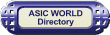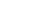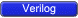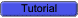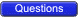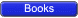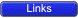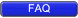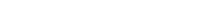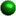Introduction Well most of the changes in Verilog 2001 are picked from other languages, like generate, configuration, file operation were from VHDL. I am just adding a list of the most commonly used Verilog 2001 changes. You may need a simulator with Verilog 2001 support for testing the examples listed below.Comma used in sensitive list In earlier version of Verilog ,we used to use 'or' to specify more than one sensitivity list element. In the case of Verilog 2001, we use comma as shown in the example below.``` 1 module comma_example(); 2 3 reg a, b, c, d, e; 4 reg [2:0] sum, sum95; 5 6 // Verilog 2k example for usage of comma 7 always @ (a, b, c, d, e) 8 begin : SUM_V2K 9 sum = a + b + c + d + e; 10 end 11 12 // Verilog 95 example for above code 13 always @ (a or b or c or d or e) 14 begin : SUM_V95 15 sum95 = a + b + c + d + e; 16 end 17 18 initial begin 19 \$monitor ("%g a=%b b=%b c=%b d=%b e=%b sum=%b sum95=%b", 20 \$time, a, b, c, d, e, sum, sum95); 21 #1 a = 1; 22 #1 b = 1; 23 #1 c = 1; 24 #1 d = 1; 25 #1 e = 1; 26 #1 \$finish; 27 end 28 29 endmodule ``` You could download file comma_example.v hereWe can use the same for edge sensitive code also, as shown in the code below.``` 1 module comma_edge_example(); 2 3 reg clk, reset, d; 4 reg q, q95; 5 6 // Verilog 2k example for usage of comma 7 always @ (posedge clk, posedge reset) 8 begin : V2K 9 if (reset) q <= 0; 10 else q <= d; 11 end 12 13 // Verilog 95 example for above code 14 always @ (posedge clk or posedge reset) 15 begin : V95 16 if (reset) q95 <= 0; 17 else q95 <= d; 18 end 19 20 initial begin 21 \$monitor ("%g clk=%b reset=%b d=%b q=%b q95=%b", 22 \$time, clk, reset, d, q, q95); 23 clk = 0; 24 reset = 0; 25 d = 0; 26 #4 reset = 1; 27 #4 reset = 0; 28 #1 d = 1; 29 #10 \$finish; 30 end 31 32 initial #1 forever clk = #1 ~clk; 33 34 endmodule ``` You could download file comma_edge_example.v hereCombinational logic sensitive list Verilog 2001 allows us to use star in sensitive list instead of listing all the variables in RHS. This removes typo mistakes and thus avoids simulation and synthesis mismatches.``` 1 module star_example(); 2 3 reg a, b, c, d, e; 4 reg [2:0] sum, sum95; 5 6 // Verilog 2k example for usage of star for combo logic 7 always @ (*) 8 begin : SUM_V2K 9 sum = a + b + c + d + e; 10 end 11 12 // Verilog 95 example for above code 13 always @ (a or b or c or d or e) 14 begin : SUM_V95 15 sum95 = a + b + c + d + e; 16 end 17 18 initial begin 19 \$monitor ("%g a=%b b=%b c=%b d=%b e=%b sum=%b sum95=%b", 20 \$time, a, b, c, d, e, sum, sum95); 21 #1 a = 1; 22 #1 b = 1; 23 #1 c = 1; 24 #1 d = 1; 25 #1 e = 1; 26 #1 \$finish; 27 end 28 29 endmodule ``` You could download file star_example.v hereWire Data type In Verilog 1995, default data type is net and its width is always 1 bit. In Verilog 2001 the width is adjusted automatically.In Verilog 2001, we can disable default data type by `default net_type none, This basically helps in catching the undeclared wires.Register Data type Register data type is called variable, as it created a lot of confusion for beginners. Also it is possible to specify an initial value for the register/variable data type. Reg data type can also be declared as signed.``` 1 module v2k_reg(); 2 3 // v2k allows to init variables 4 reg a = 0; 5 // Here only last variable is set to 0, i.e d = 0 6 // Rest b, c are set to x 7 reg b, c, d = 0; 8 // reg data type can be signed in v2k 9 // We can assign with signed constants 10 reg signed [7:0] data = 8'shF0; 11 12 // Function can return signed values 13 // Its ports can contain signed ports 14 function signed [7:0] adder; 15 input a_in; 16 input b_in; 17 input c_in; 18 input signed [7:0] data_in; 19 begin 20 adder = a_in + b_in + c_in + data_in; 21 end 22 endfunction 23 24 endmodule ``` You could download file v2k_reg.v hereNew operators Verilog 2001 introduced two new operator that are of interest to designers. They are<<< : Shift left, to be used on signed data type >>> : shift right, to be used on signed data type ** : exponential power operator.Signed shift operator``` 1 module signed_shift(); 2 3 reg [7:0] unsigned_shift; 4 reg [7:0] signed_shift; 5 reg signed [7:0] data = 8'hAA; 6 7 initial begin 8 unsigned_shift = data >> 4; 9 \$display ("unsigned shift = %b", unsigned_shift); 10 signed_shift = data >>> 4; 11 \$display ("signed shift = %b", signed_shift); 12 unsigned_shift = data << 1; 13 \$display ("unsigned shift = %b", unsigned_shift); 14 signed_shift = data <<< 1; 15 \$display ("signed shift = %b", signed_shift); 16 \$finish; 17 end 18 19 endmodule ``` You could download file signed_shift.v herePower operator``` 1 module power_operator(); 2 3 reg [3:0] base = 2; 4 reg [5:0] exponent = 1; 5 reg [31:0] result = 0; 6 7 initial begin 8 \$monitor ("base = %d exponent = %d result = %d", base, exponent, result); 9 repeat (10) begin 10 #1 exponent = exponent + 1; 11 end 12 #1 \$finish; 13 end 14 15 always @ (*) 16 begin 17 result = base ** exponent; 18 end 19 20 endmodule ``` You could download file power_operator.v herePort Declaration Verilog 2001 allows port direction and data type in the port list of modules as shown in the example below.``` 1 module ansiport_example(); 2 3 reg read,write = 0; 4 reg [7:0] data_in = 0; 5 reg [3:0] addr = 0; 6 wire [7:0] data_v95, data_notype, data_ansi; 7 8 initial begin 9 \$monitor ( 10 "%g rd=%b wr=%b addr=%b data_in=%h data_v95=%h data_notype=%h data_ansi=%h" 11 , \$time, read, write, addr, data_in, data_v95, data_notype, data_ansi); 12 #1 read = 0; // why only for read ? 13 #3 repeat (16) begin 14 data_in = \$random; 15 write = 1; 16 #1 addr = addr + 1; 17 end 18 write = 0; 19 addr = 0; 20 #3 repeat (16) begin 21 read = 1; 22 #1 addr = addr + 1; 23 end 24 read = 0; 25 #1 \$finish; 26 end 27 28 memory_v95 U (read, write, data_in, addr, data_v95); 29 memory_ansi_notype W (read, write, data_in, addr, data_notype); 30 memory_ansi V (read, write, data_in, addr, data_ansi); 31 32 endmodule 33 // Verilog 95 code 34 module memory_v95 ( read, write, data_in, addr, data_out); 35 input read; 36 input write; 37 input [7:0] data_in; 38 input [3:0] addr; 39 output [7:0] data_out; 40 41 reg [7:0] data_out; 42 reg [7:0] mem [0:15]; 43 44 always @ (*) 45 begin 46 if (write) mem[addr] = data_in; 47 end 48 49 always @ (read, addr) 50 begin 51 if (read) data_out = mem[addr]; 52 end 53 54 endmodule 55 56 // Verilog 2k with notype in port list 57 module memory_ansi_notype ( 58 input read, 59 input write, 60 input [7:0] data_in, 61 input [3:0] addr, 62 output [7:0] data_out 63 ); 64 reg [7:0] mem [0:15]; 65 66 always @ (*) 67 begin 68 if (write) mem[addr] = data_in; 69 end 70 71 assign data_out = (read) ? mem[addr] : 0; 72 73 endmodule 74 75 // Verilog 2k with width and data type listed 76 module memory_ansi ( 77 input wire read, 78 input wire write, 79 input wire [7:0] data_in, 80 input wire [3:0] addr, 81 output reg [7:0] data_out 82 ); 83 84 reg [7:0] mem [0:15]; 85 86 always @ (*) 87 begin 88 if (write) mem[addr] = data_in; 89 end 90 91 always @ (read, addr) 92 begin 93 if (read) data_out = mem[addr]; 94 end 95 96 endmodule ``` You could download file ansiport_example.v here